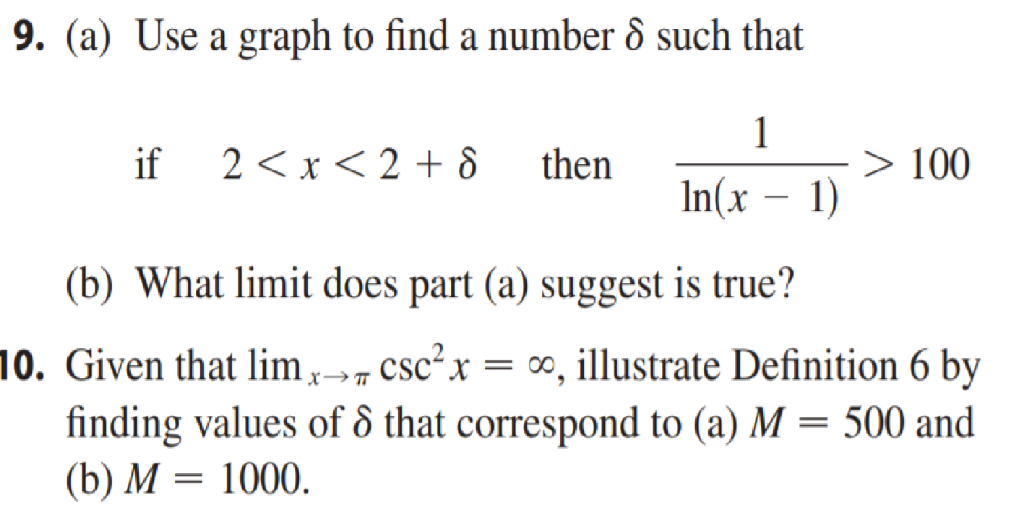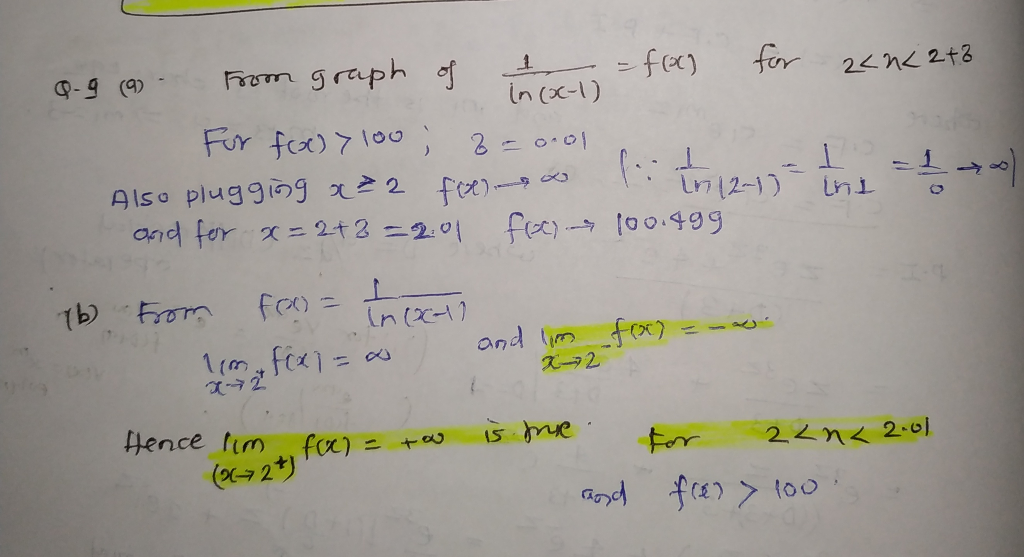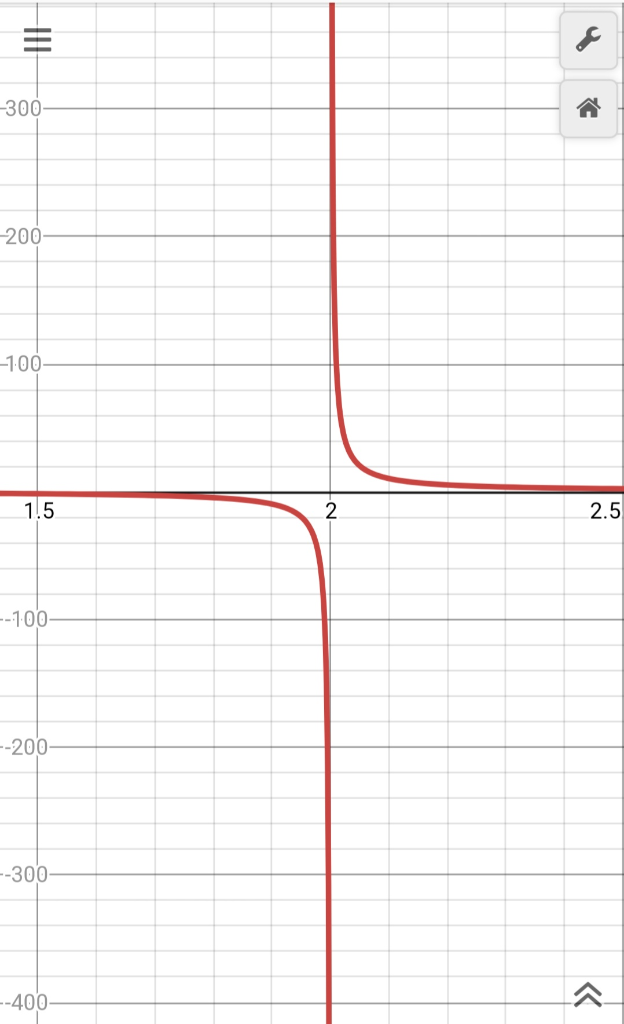# 9. (a) Use a graph to find a number δ such that 2<x<2+δ if then In(x - 1) (b) What limit does part (a) suggest is...9. (a) Use a graph to find a number δ such that 2Hope it helps you out.

Thanks

Note: Q. 10 need, definition 6 as reference, which is missing.

##### Add Answer of: 9. (a) Use a graph to find a number δ such that 2<x<2+δ if then In(x - 1) (b) What limit does part (a) suggest is...
More Homework Help Questions Additional questions in this topic.